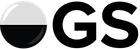# Small change to scatterplot

#1

Hiho.

I know it’s mostly a gimmick, but maybe it’s a good idea to add a title and a legend to the game-offer scatterplot.
I imagine most new users (and yours truly sometimes) look at it in bewilderment.Thought of this because I saw a giant violet square (what do differences in square size indicate?) and I know that grey means I can’t accept the offer, green means I can… but what does violet do?

2 Likes
#2

If I understand it correct, there are 7 different colors:
blue (#4140FF, #58E0FF), green (#00aa30, #00ff00), magenta (#f000d0, #ff60dd), cyan (#009090, #00ffff), orange (#d06000, #ff9000), red (#E25551, #bbb) and gray (#bbbbbb, #bbb). In parenthesis are the htmll color-codes of the fill and border colors (I cannot use colors on forum posts)

They are used as follows:

• blue for “live games”. I think this isn’t used at the moment. Has someone seen that sometimes?
• green ranked 19x19 games one can accept.
• magenta ranked 13x13 games one can accept.
• cyan ranked 9x9 games one can accept.
• orange unranked games one can accept (any board size).
• red own challenges.
• gray not acceptable challenges.

If there is more than one open challenge for a point (time_per_move, rank) in the seek graph, the rectangle is bigger. (don’t trust the colors there, the color corresponds to at least 1 of the games, not necessarily all of them. Gray or red are only used if all of the games are either color).

source code
``````    redraw() {{{
let ctx = this.canvas.getContext("2d");
let w = this.canvas.width();
let h = this.canvas.height();
let padding = this.padding;
let blitz_line = Math.round(SeekGraph.blitz_line_ratio * (w - padding) + padding);
let live_line = Math.round(SeekGraph.live_line_ratio * (w - padding) + padding);

ctx.clearRect(0, 0, w, h);
this.drawAxes();

let plot_ct = {};
this.challenge_points = {};

let sorted = [];
for (let id in this.challenges) {
sorted.push(this.challenges[id]);
}
sorted.sort((A, B) => {
if (A.eligible && !B.eligible) { return 1; }
if (!A.eligible && B.eligible) { return -1; }

if (A.user_challenge && !B.user_challenge) { return 1; }
if (!A.user_challenge && B.user_challenge) { return -1; }

if (A.ranked && !B.ranked) { return 1; }
if (!A.ranked && B.ranked) { return -1; }

return B.challenge_id - A.challenge_id;
});
if (this.show_live_games) {
for (let id in this.live_games) {
sorted.push(this.live_games[id]);
}
}

/* Plot our challenges */
for (let j = 0; j < sorted.length; ++j) {
let C = sorted[j];

let rank_ratio = (Math.min(MAX_RATIO, (C.rank + 1) / 40));

let time_ratio = 0;
let last_tpm = 0;
for (let i = 0; i < SeekGraph.time_columns.length; ++i) {
let col = SeekGraph.time_columns[i];
if (C.time_per_move <= col.time_per_move) {
if (C.time_per_move === 0) {
time_ratio = col.ratio;
break;
}

let alpha = (C.time_per_move - last_tpm) / (-last_tpm + col.time_per_move);
time_ratio = lerp(time_ratio, col.ratio, alpha);
break;
}
last_tpm = col.time_per_move;
time_ratio = col.ratio;
}

let cx = Math.round(padding + ((w - padding) * time_ratio));
let cy = Math.round(h - (padding + ((h - padding) * rank_ratio)));

C.cx = cx;
C.cy = cy;

let plot_ct_pos = time_ratio + "," + rank_ratio;
if (!(plot_ct_pos in plot_ct)) {
plot_ct[plot_ct_pos] = 0;
}
let ct = ++plot_ct[plot_ct_pos];

/* Draw */
let d = this.square_size;
let sx = cx - d / 2 + 0.5;
let sy = cy - d / 2 + 0.5;
ctx.save();
ctx.beginPath();
ctx.strokeStyle = "#000";
if (C.live_game) {
ctx.fillStyle = "#4140FF";
ctx.strokeStyle = "#58E0FF";
} else if (C.eligible) {
if (C.ranked) {
if (C.width === 19) {
ctx.fillStyle = "#00aa30";
ctx.strokeStyle = "#00ff00";
} else if (C.width === 13) {
ctx.fillStyle = "#f000d0";
ctx.strokeStyle = "#ff60dd";
} else {
ctx.fillStyle = "#009090";
ctx.strokeStyle = "#00ffff";
}
} else {
ctx.fillStyle = "#d06000";
ctx.strokeStyle = "#ff9000";
}
ctx.fillRect(sx, sy, d, d);
} else if (ct === 1) {
if (C.user_challenge) {
ctx.fillStyle = "#E25551";
ctx.strokeStyle = "#bbb";
} else {
ctx.fillStyle = "#bbbbbb";
ctx.strokeStyle = "#bbb";
}
ctx.fillRect(sx, sy, d, d);
}
if (ct > 1) {
let cc = Math.min((this.width < 200 ? 2 : 4), ct - 1);
sx -= cc;
sy -= cc;
d += cc * 2;
}
if (!C.eligible) {
ctx.strokeStyle = "#bbb";
}
if (C.eligible || C.live_game) {
ctx.strokeRect(sx, sy, d, d);
}
ctx.restore();
}

//console.log("Redrawing seekgraph");
}}}
``````

https://github.com/online-go/online-go.com/blob/devel/src/components/SeekGraph/SeekGraph.tsx

7 Likes
Play screen grid colours
OGS Match Scatterplot
#3

Aye, that’s a lot more than I recall. Warrants a legend, I think. Thanks for the digging.4 Likes
#4

Does anyone actually use scatterplot to pick challenges? I tried once and found it almost useless.

I think it would be nice to have those informations in the challenge list below in a more intuitive way than just text. Especially the “ranked” option, board size and timesetting.

It actually would be very good to have a gradient for timesetting, in order to highlight when a “correspondence” game has a very fast timesetting (e.g. canadian 24h x 10 moves) or very slow (fisher 21d + 1d per move).

I’m not very good at charts so, trying to figure how to show all those coloured informatiions in the text table ended up in a sort of clown vomit. But I’m sure someone could find an appealing way to do it.

2 Likes
#5

I have never used it.

1 Like
#6

Now that I know what the colors mean, I’ll use it more.

2 Likes
#7

I think the place where it’s useful is in chat. If you are hanging in chat and see a blip in the area you are interested in (blitz 13x13 you can accept say) then you can rush over to the Play page and accept.

It’s reasonably useless on the play page because you can scan the open games you can accept in one quick look I think.

2 Likes